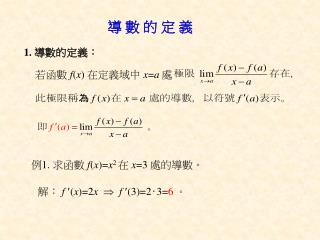DownloadDownload Presentation1. 導數的定義：

1. 導數的定義：

Télécharger la présentation1. 導數的定義：

- - - - - - - - - - - - - - - - - - - - - - - - - - - E N D - - - - - - - - - - - - - - - - - - - - - - - - - - -
Presentation Transcript

1. 導 數 的 定 義 1. 導數的定義： 若函數 f(x) 在定義域中 x=a 處 例1. 求函數 f(x)=x2 在 x=3 處的導數。 解：f (x)=2x f (3)=2‧3=6。

2. 例2.試問函數 在 x=0 處的導數是否存在﹖ y x O

3. 2. 導數的另一個定義： 令 h=xa ，則當 h 趨近 0 時，x 也會趨近 a。 因此，函數 f(x) 在 x=a 處的導數也可表為

4. 範例：設 f(x) = x2，求 f (x)。 馬上練習. 設

5. 例3. 設函數 f(x)=2x33x25x+4，求(1) f (x) (2) f (x) 。 解：(1) f (x)=6x2 6x5。 (2) f (x)=12x6。 練習. 設函數 f(x)=2x4+x33x2+4x8，求(1) f (x)(2) f (x) 。 解：(1) f (x)=8x3 +3x26x+4。 (2) f (x)=24x2+6x6。

6. 例4.(1) 設f(x)=(x22x1)10，求f (2) 。 (2) 設 f(x)=(x2x+1)10(x2)20，求 f (1)。 解：(1) f (x)=10(x22x1)9‧(2x2)。 f (2)=10(1)9‧(2)= 20。 (2) f (x)=[10(x2x+1)9‧(2x1)](x2)20 +(x2x+1)10[20(x2)19]‧1 f (1)=[10‧19‧1](1)20+110 [20(1)19] =1020= 10。

7. 練習. (1) 設 f(x)=(x2+x1)10，求f (1)。 (2) 設 f(x)=(x1)10(2x5)30，求 f (2)。 解：(1) f (x)=10(x2+x1)9‧ (2x+1)。 f (1)=10‧19‧3=30。 (2) f (x)=[10(x1)9‧1](2x5)30 +(x1)10[30(2x5)29]‧2 f (2)=[10‧19](1)30+110 [30(1)29]‧2 =1060= 50。

8. 例5. 已知 P(1,2) 為二次函數 f(x)= x2+3 的圖形上一點， y 求以 P 為切點的切線方程式。 解： f (x)= 2x， y=x2+3 P(1,2)  x O 切線 L 練習已知 P(2,3) 為二次函數 f(x)=x2x+1 的圖形上一點， 求以 P 為切點的切線方程式。 Ans：f (x)=2x1， f (2)=3  所求 3xy=3。

9. 例6. 設函數 f(x)=x3+2x23x+2，求： (1) f (x)(2) f (1) (3) 函數 f(x) 的圖形上以點 (1,2) 為切點之切線方程式。 解：(1) f (x)=3x2+4x3。 (2) f (1)=3+43=4。

10. 練習. 求函數 f(x)=x32x3 的圖形上 以點 (2,1) 為切點之切線方程式。 解： f (x)=3x22 , f (2)=122=10。 類題.求在函數 y=f(x)=(12x)6(2x3) 的圖形上， 以點 P(1,1) 為切點的切線方程式﹒ 解： f (x)=[6(12x)5‧(2)](2x3) +(12x)6‧2 f (1)= 10。

11. 例7. 已知在函數 f(x)=2x3+3x29x+5 的圖形上， 以點 P 為切點的切線斜率為 3，求切點 P 的坐標。 解： f (x)=6x2+6x9。 若 f (x)= 3  6x2+6x9 =3  x=1 或 2 。 (1)當 x=1  y=2+39+5=1  P(1,1)。 (2)當 x= 2  y= 16+12+18+5=19  P( 2,19)。

12. 練習. 已知在函數 f(x)=x2+x2 的圖形上， 以點 P 為切點的切線斜率為 5，求切點 P 的坐標。 解： f (x)=2x+1， 若 f (x)=5  2x+1=5  x=2。 當 x=2  y=4+22=4  P(2,4)。

13. 例8. 已知 P(2,2) 為二次函數 f(x)=x22x+2 的圖形外一點， 求通過 P 點的切線方程式。 y y=x22x+2 解：設切點A(a , a22a+2)  A(a,a22a+2) ∵f (x)=2x2 ∴ 切線斜率=f (a)=2a2，  切線 L 過 P 點的切線 L：y(2)=(2a2)(x2) x O A在 L上： a22a+2(2)=(2a2)(a2)  P(2, 2) 整理得a24a=0  a=4 或 0 。 (1)切點(4,10)， f (4)=6  切線 L：6xy=14。 (2)切點(0,2)，f (0)= 2  切線 L：2x+y=2。

14. 練習. 已知 P(1,1) 為二次函數 f(x)= x2+1 的圖形外一點， 求通過 P 點的切線方程式。 切線 L 解：設切點A(a ,a2+1) ∵f (x)= 2x P(1,1)   ∴ 切線斜率=f (a)= 2a， A(a,a2+1)  過 P 點的切線 L：y1=(2a)(x1) y=x2+1 A在 L上： a2+11=(2a)(a1) 整理得a22a=0  a=2 或 0 。 (1)切點(2,3)， f (2)= 4  切線 L：4x+y=5。 (2)切點(0,1)，f (0)=0  切線 L：y=1。

15. 例9. 如下圖所示，A與B為二次函數f(x)=x2圖形上的相異兩點， 自 AB 弦的中點 M 作鉛直線交函數 f(x)圖形於 C 點， 試證：以 C 點為切點的切線與直線 AB 平行。 y y=x2 解：設A(a , a2)，B(b , b2) A(a,a2)  M   B(b,b2)  C x O 所以直線 AB 的斜率等於過 C 點的切線斜率。

16. 丙、導數在運動學上的意義 設 f(x) 表示一運動質點的位移函數。 (1) 若導函數 f (x) 存在，則此質點的速度函數為 f (x)。 (2) 若第二階導函數 f (x) 存在， 則此質點的加速度函數為 f (x)。

17. 例10. 已知有一運動質點的位移函數是 f(x)=50x4.9x2， 求此質點在時刻 x=2 的瞬時速度及瞬時加速度。 解： f (x)=509.8x ， f (x)= 9.8 ， 在時刻 x=2 的瞬時速度=f (2)=5019.6=30.4。 在時刻 x=2 的瞬時加速度=f (2)= 9.8。

18. 練習. 已知小明參加一百公尺比賽時﹐從起跑到抵達終點 這段時間內，測得其經 t 秒後前進的距離為 公尺，求下列各題： (1) 小明在 t=3 秒時的瞬時速度及瞬時加速度。 (2) 當小明衝過終點線時的瞬時速度。